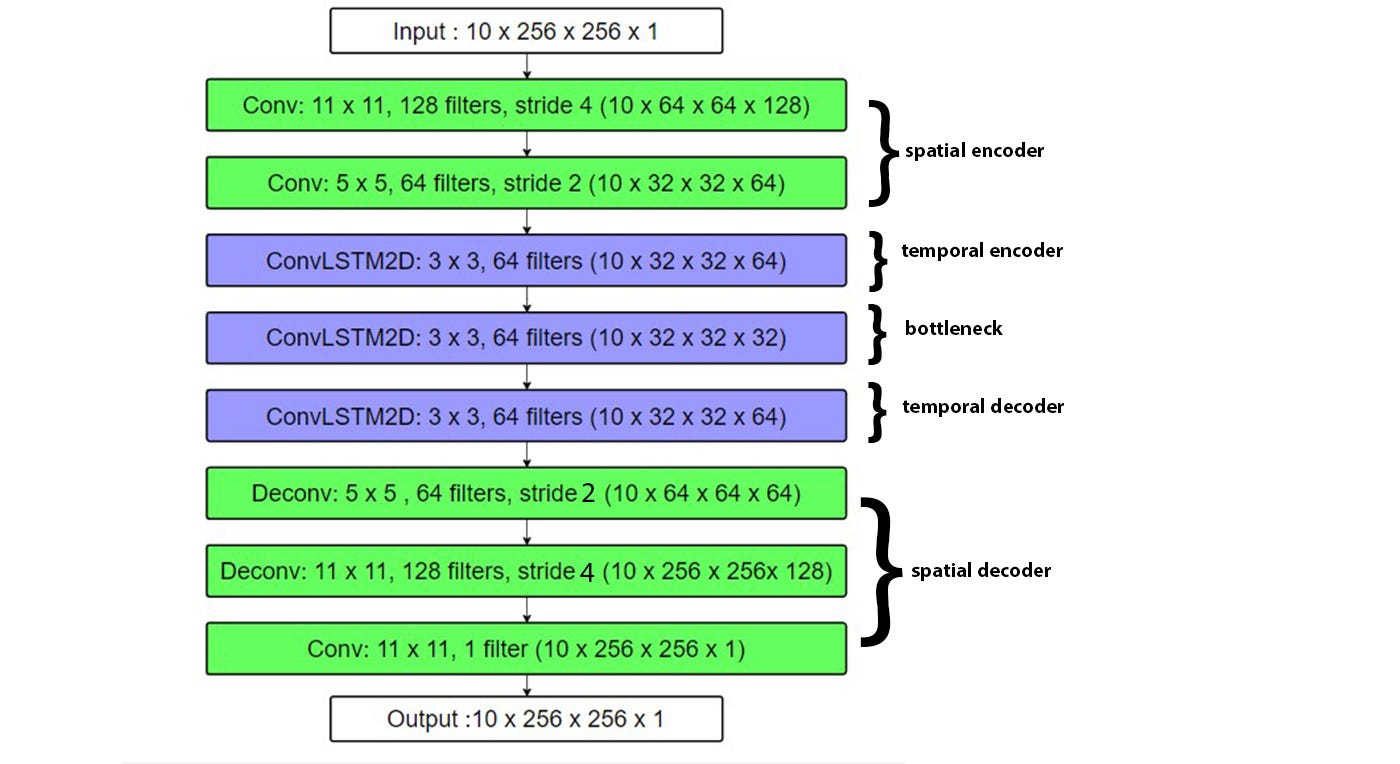Intel® Distribution of OpenVINO™ Toolkit
Community support and discussions about the Intel® Distribution of OpenVINO™ toolkit, OpenCV, and all things computer vision-related on Intel® platforms.
5961 Discussions

## OpenVino Model Optimizer - Can't find the right weightsBeginner
261 Views

Hello!

I'm trying to implement a LSTM model for anomaly detection in OpenVino

I got the Keras model from a blog post then converted it to a frozen Tensorflow model following another, but I'm stuck at the "optimising the model and converting it to IR" part.

I'm getting that error:

[ ERROR ]  Shape [ -1  10 256 256   1] is not fully defined for output 0 of "time_distributed_1_input_4". Use --input_shape with positive integers to override model input shapes.
[ ERROR ]  Cannot infer shapes or values for node "time_distributed_1_input_4".

I understand that we need to specify the batch size, but the original model seems to have a flexible one, am I forced to fix it in the code and retrain it? Or is there a workaround?

Thanks a lot.

Shape of the model:Here is the part related to model creation:

```    seq = Sequential()
seq.add(TimeDistributed(Conv2D(128, (11, 11), strides=4, padding="same"), batch_input_shape=(None, 10, 256, 256, 1)))
# # # # #
# # # # #
print(seq.summary())
seq.compile(loss='mse', optimizer=tf.keras.optimizers.Adam(lr=1e-4, decay=1e-5, epsilon=1e-6))
seq.fit(training_set, training_set,
batch_size=Config.BATCH_SIZE, epochs=Config.EPOCHS, shuffle=False)```

Here are the errors I got in the workbench when removing a dimension, or replacing it with 190 and 200: